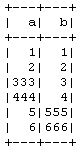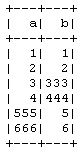# Merge two DataFrames in PySpark

• Last Updated : 04 May, 2022

In this article, we will learn how to merge multiple data frames row-wise in PySpark. Outside chaining unions this is the only way to do it for DataFrames. The module used is pyspark :

Spark (open-source Big-Data processing engine by Apache) is a cluster computing system. It is faster as compared to other cluster computing systems (such as Hadoop). It provides high-level APIs in Python, Scala, and Java. Parallel jobs are easy to write in Spark. We will cover PySpark (Python + Apache Spark) because this will make the learning curve flatter. To install Spark on a linux system, follow this. To run Spark in a multi–cluster system, follow this.

To do our task we are defining a function called recursively for all the input dataframes and union this one by one. To union, we use pyspark module:

• Dataframe union() – union() method of the DataFrame is employed to mix two DataFrame’s of an equivalent structure/schema. If schemas aren’t equivalent it returns a mistake.
• DataFrame unionAll() – unionAll() is deprecated since Spark “2.0.0” version and replaced with union().

Note: In other SQL’s, Union eliminates the duplicates but UnionAll combines two datasets including duplicate records. But, in spark both behave an equivalent and use DataFrame duplicate function to get rid of duplicate rows.

At the last call, it returns the required resultant dataframe. The following code represents the logic behind our solution to the given problem.

## Python3

 `from` `functools ``import` `reduce`  `# For Python 3.x``from` `pyspark.sql ``import` `DataFrame` `def` `unionAll(``*``dfs):``    ``return` `reduce``(DataFrame.unionAll, dfs)` `unionAll(td2, td3, td4, td5, td6, td7, td8, td9, td10)`

What takes place is that it takes all the objects that you handed as parameters and reduces them the usage of unionAll (this limit is from Python, no longer the Spark minimize even though they work similarly) which sooner or later reduces it to one DataFrame.

If rather of DataFrames are ordinary RDDs you can bypass a listing of them to the union feature of your SparkContext

### Examples:

Sometimes, when the dataframes to combine do not have the same order of columns, it is better to df2.select(df1.columns) in order to ensure both df have the same column order before the union.

## Python3

 `import` `functools` `def` `unionAll(dfs):``    ``return` `functools.``reduce``(``lambda` `df1, df2: df1.union(df2.select(df1.columns)), dfs)`

The reduce(fun,seq) function is used to apply a particular function passed in its argument to all the list elements mentioned in the sequence passed along. This function is defined in functools module.

Now, let’s understand the whole process with the help of some examples.

Example 1:

In this example, we create dataframes with columns ‘a’ and ‘b’ of some random values and pass all these three dataframe to our above-created method unionAll() and get the resultant dataframe as output and show the result.

## Python3

 `# import modules``from` `pyspark.sql ``import` `SparkSession``import` `functools` `# explicit function``def` `unionAll(dfs):``    ``return` `functools.``reduce``(``lambda` `df1, df2: df1.union(df2.select(df1.columns)), dfs)`  `spark ``=` `SparkSession.builder.getOrCreate()``df1 ``=` `spark.createDataFrame([[``1``, ``1``], [``2``, ``2``]], [``'a'``, ``'b'``])` `# different column order.``df2 ``=` `spark.createDataFrame([[``3``, ``333``], [``4``, ``444``]], [``'b'``, ``'a'``])``df3 ``=` `spark.createDataFrame([[``555``, ``5``], [``666``, ``6``]], [``'b'``, ``'a'``])` `unioned_df ``=` `unionAll([df1, df2, df3])``unioned_df.show()`

Output:Example 2:

In this example, we create dataframes with columns ‘a’ and ‘b’ of some random values and pass all these three dataframe to our newly created method unionAll() in which we are not focusing on the names of the columns. We are just doing union the input dataframe to the next dataframe and get the resultant dataframe as output and show the result.

## Python3

 `# import modules``from` `functools ``import` `reduce` `from` `pyspark.sql ``import` `DataFrame``from` `pyspark.sql ``import` `SparkSession` `# explicit functions``def` `unionAll(``*``dfs):``    ``return` `reduce``(DataFrame.unionAll, dfs)`  `spark ``=` `SparkSession.builder.getOrCreate()` `df1 ``=` `spark.createDataFrame([[``1``, ``1``], [``2``, ``2``]], [``'a'``, ``'b'``])` `# different column order.``df2 ``=` `spark.createDataFrame([[``3``, ``333``], [``4``, ``444``]], [``'b'``, ``'a'``])``df3 ``=` `spark.createDataFrame([[``555``, ``5``], [``666``, ``6``]], [``'b'``, ``'a'``])` `unionAll(``*``[df1, df2, df3]).show()`

Output:Important:

• Notice how values for columns a, b are mixed up in here – that’s because when performing a union the order the columns isn’t matching.
• since both columns are of type string we get no error.
• In order to perform a valid union order should match across all DataFrames.
another option is using UnionByName

My Personal Notes arrow_drop_up